# Karnaugh Map Solver

To use the karnaugh solver, select the option of SOP or POS, select a name for the output, enter the variables, input the minterms and the don’t cares, and click calculate## Karnaugh map solver

The Karnaugh map solver will find the k-map with the answer of Sum of products and product of sums in three types of formats.

1. Generic solution [using (+),(.),(’)]
2. VHDL solution [using AND, NOT, and OR]
3. Verilog solution [using (~),(|), and (&)]

Get the Karnaugh map for 2,4,6 and even 10 variables in the karnaugh map calculator.

## What is the karnaugh map?

Developed by the physicist Maurice Karnaugh, this map finds the solution of binary expressions in picture form and simplifies them without using any law or theorem.

## Formula for karnaugh map:

The map is formed under rules but the basic point of the map is its formula.

2n where n represents the number of variables. This formula will tell about the number of cells/squares to be drawn.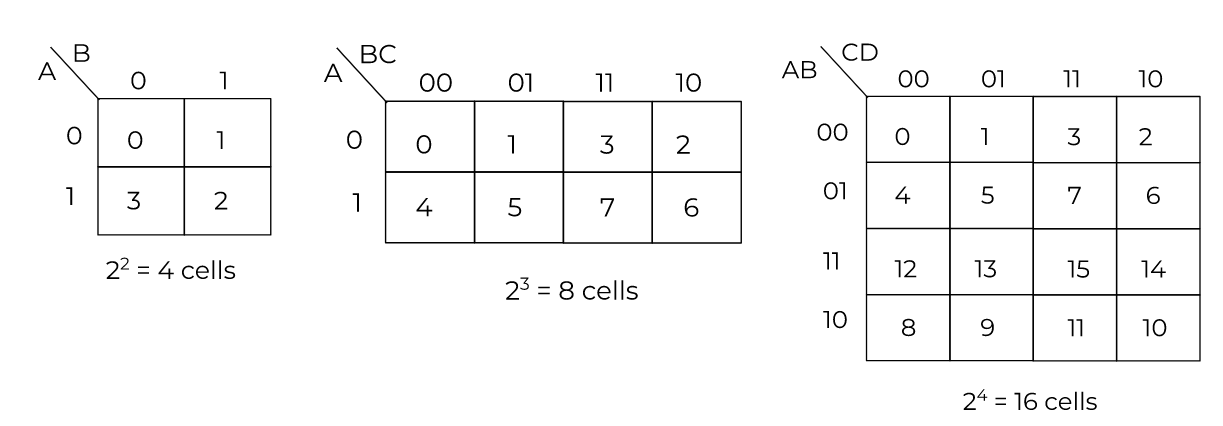The digits inside the cell represent the cell number. They are to be memorized. A trick to remember these numbers is by multiplying the row and column binary number for that cell and finding its decimal representation.

For example, 5 is represented as 101 in binary. If you locate cell no.5 in the map and multiply its column and row number, it will give 101 as well.

### Rules for k-map:

1. Always use “1” to represent a minterm in a cell.
2. Make horizontal or vertical groups. Diagonal grouping is not allowed.
3. One cell can be a part of two or more groups at the same time. Overlapping is allowed.
4. Make groups in pairs, quads, or octets. In short, as large as possible. Try to make an octet. If not possible, look for a quad and then a pair.

Below are examples of pair and quad grouping.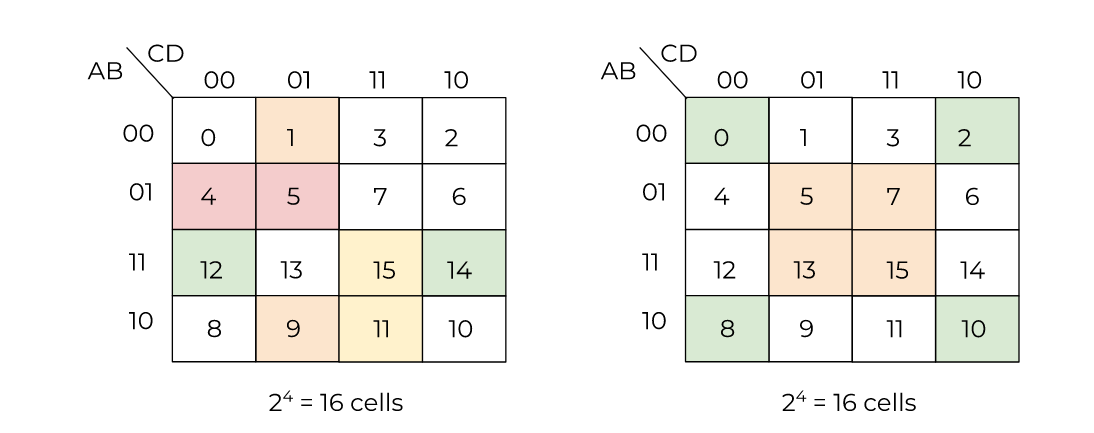## How to make a karnaugh map (k-map)?

Look for the number of variables. Use the number as the exponent of the 2 and calculate the required cell numbers. Represent the minterms by 1 after locating the cells.

Example:

Make the k-map from the following expression and form groups.

F (A,B,C,D) = (4,12,6,14,8,10)

Solution:

Step 1: Find the number of cells.

There are 4 variables. Using 4 as the power of 2, we get:

24 = 16

Step 2: Make cells.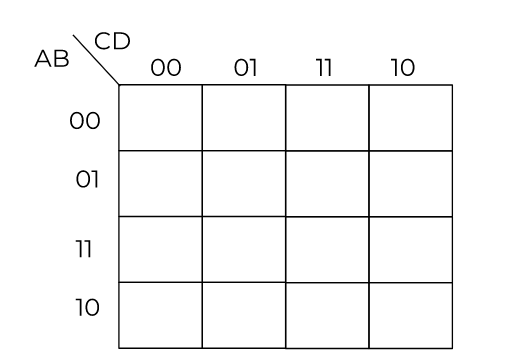Step 3: Locate the cells and place one in them.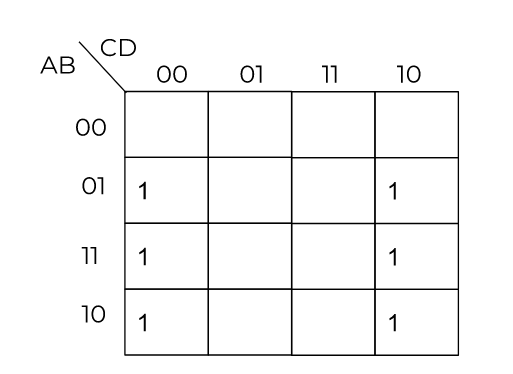Step 4:  Make groups.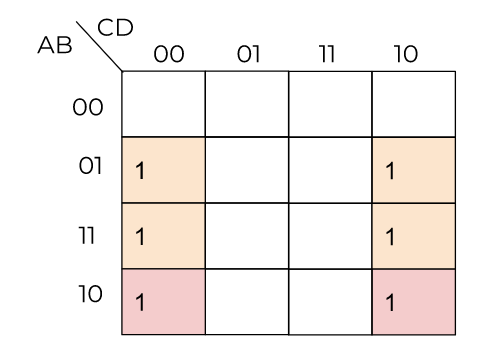A quad and a pair are formed.

## SOP and POS of karnaugh map:

Sum of products and product of sums are methods of representing boolean expressions. Both have their uses depending on the situation.

### Key points:

Minterms are values giving the output 1. Minterms are represented in the SOP.

Max terms result in zeros and include all the remaining cells except don’t cares. These are used in POS.

Don’t care are cells represented by “x”. They are often used to complete groups.

A pair of 1 and 0 is canceled.

### Sum of products:

All 0’s mean complement of the variable. All ones mean empty or normal variables. Variables of a group are separated by a dot (multiplication) and groups are separated by a plus (addition).

Example:

Find the SOP of this boolean expression.

F(A,B,C,D) =  m (0,6,8,13,14)
= d (2,4,10)

Solution:

Step 1: Make k-map.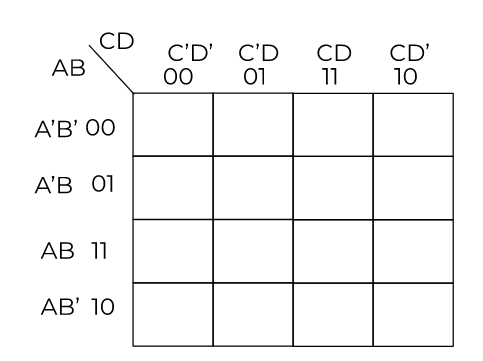Step 2: Place the minterms and don’t cares.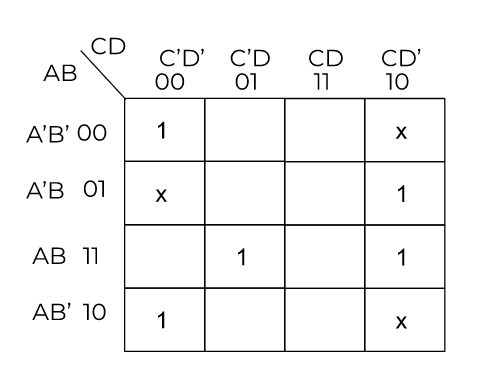Step 3: Make groups.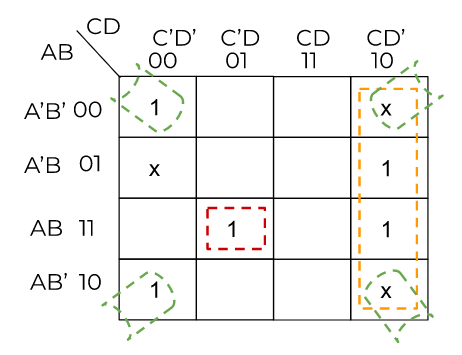Two quads and a singleton are formed. As you can see the second quad used the don’t care values as 1.

Step 4: Write the binary value of groups.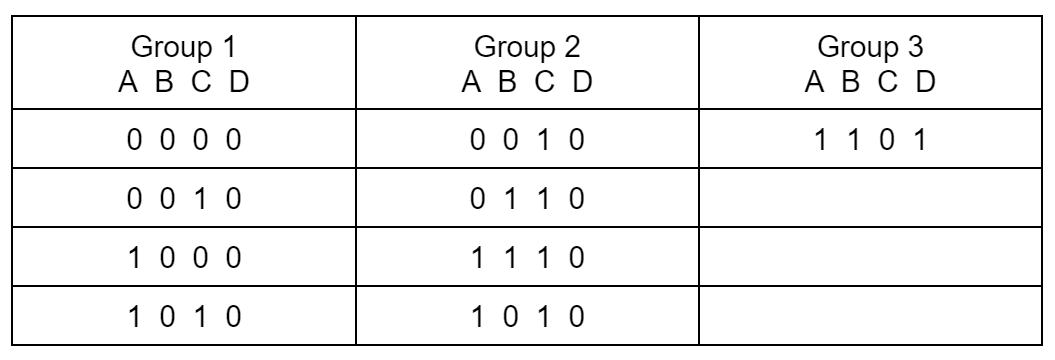Step 5: Write the sum of products.

F =    (B’ . D’) +   (C . D’) + (A . B . C’ . D)

### Product of sums:

All 1’s mean complement of the variable. All zeros mean empty or normal variable. Variables of a group are separated by a sum (addition) and groups are separated by a dot (multiplication).

Example:

For the previous example, find the product of the sums.

Solution:

Step 1: After making the k-map as before, enter the 0’s as maxterms. Also, invert the complements.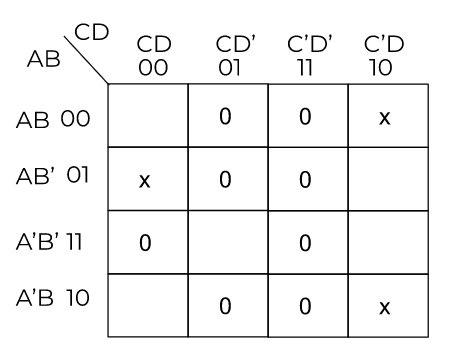Step 2: Make groups.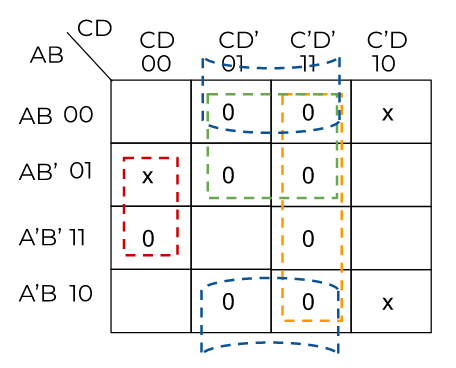Step 3: Write the binary values and cancel the 1’s and 0’s.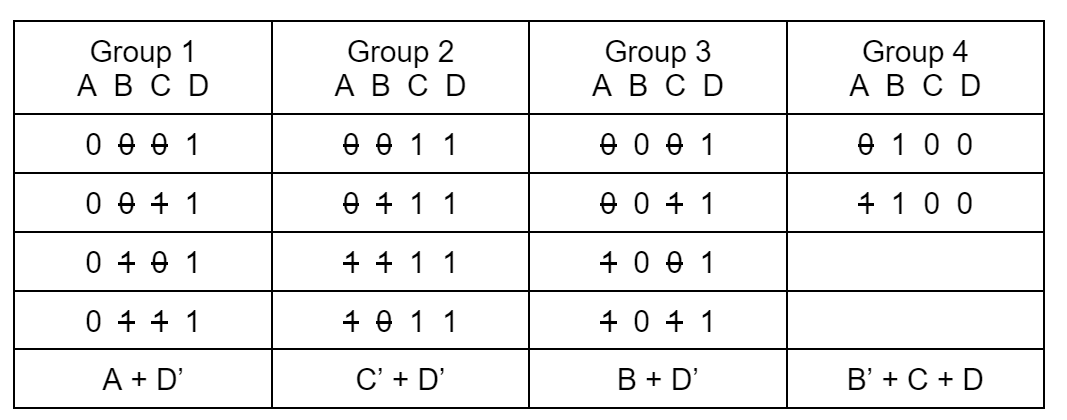Step 4: Write the Product of sums.
F = (A + D’)(C’ + D’)(B + D’)(B’ + C + D)